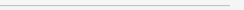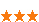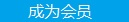您可以捐助，支持我们的公益事业。 1元 10元 50元 认证码：必填求知 文章 文库 Lib 视频 Code iProcess 课程 角色 咨询 工具 火云堂 讲座吧 建模者要资料订阅捐助
Swift中的let和var背后的编程模式854 次浏览     评价： 好 中 差

Swift中有两种声明“变量”的方式，这两种方式分别使用let和var这两个关键字。这应该是借鉴了Scala，因为它们和Scala的val和var有相同的作用。let被用于声明不变量，var被用于声明变量。不变量的值一旦被定义就不能再改变，变量则可以在声明之后被随意赋值。

“缺省的是变量，如果需要不变量，请显式说明”。这是大多数命令式编程语言对变量和不变量的处理方法。这很自然。因为这类语言的设计中，大多数情况下使用的是变量，不变量只是在特殊情况下才需要。Swift（和Scala一样）则对这种设计做出了修改。从缺省是变量，转变为认为变量和不变量的地位是平等的。不变量应该更多被提倡和使用。在Swift的语法中，对这种设计思想的体现是：在定义一个和值关联的名字时，需要明确地使用var或let说明它是变量还是不变量。

Swift，和Java，C，Objective-C等语言相比，为何会有这种对待不变量的观点的变化呢？

Swift受到了函数式编程的影响，强化了不变量在语言中位置，鼓励不变量的使用。

 `f(x) = 2*x + x * x`

 `func foo(x: Int) -> Int { return 2*x + x * x}`

 `func bar(x: Int) -> Int { var y = 2 * x y = y + x * x return y}`

Swift中提供let声明不变量，更为重视不变性，明确鼓励在更多的场合使用不变量。这都是受函数式编程中变量的不变性的影响。后面会讨论Swift为何会受到这种影响。

 `struct Point { let x: Double let y: Double}` let p1 = Point(x: 1, y: 2) let p2 = Point(x: 1, y: 2)

 `struct Point { var x: Double var y: Double}` var p1 = Point(x: 1, y: 2) var p2 = Point(x: 1, y: 2)

 `class Point { var x: Double var y: Double` init(x: Double, y: Double) { self.x = x self.y = y } } var p1 = Point(x: 1, y: 2) var p2 = Point(x: 1, y: 2) var p3 = p1 p3.x = 2

 `struct Point { var x: Double var y: Double}` var p1 = Point(x: 1, y: 2) var p2 = Point(x: 1, y: 2) var p3 = p1

 `func factorial(n: Int) -> Int { var product = 1 var i = 1 while i <= n { product = i * product i = i + 1 }` return product }

 `func factorial(n: Int) -> Int { if n == 0 { return 1 } return n * factorial(n - 1)}`

 `class account { var balance: Double` init(balance: Double) { self.balance = balance } func withdraw(amount: Double) { let newBalance = self.balance - amount // #1 self.balance = newBalance // #2 } func deposit(amount: Double) { let newBalance = self.balance + amount self.balance = newBalance } } let george = account(balance: 100) let paul = george george.withdraw(10) paul.withdraw(20)

Swift中有两个声明变量的关键字：let和var。这两个关键字背后存在着两种截然不同的编程思想：函数式编程和命令式编程。Swift对这两种编程思想进行了融合：它允许你使用引入赋值所带来的简单直观的建模方854 次浏览  评价: 好 中 差订阅捐助
 相关文章 手机软件测试用例设计实践 手机客户端UI测试分析 iPhone消息推送机制实现与探讨 Android手机开发（一）
 相关文档 Android_UI官方设计教程 手机开发平台介绍 android拍照及上传功能 Android讲义智能手机开发
 相关课程 Android高级移动应用程序 Android系统开发 Android应用开发 手机软件测试

 android人机界面指南 Android手机开发（一） Android手机开发（二） Android手机开发（三） Android手机开发（四） iPhone消息推送机制实现探讨 手机软件测试用例设计实践 手机客户端UI测试分析 手机软件自动化测试研究报告

 Android高级移动应用程序 Android应用开发 Android系统开发 手机软件测试 嵌入式软件测试 Android软、硬、云整合

 领先IT公司 android开发平台最佳实践 北京 Android开发技术进阶 某新能源领域企业 Android开发技术 某航天公司 Android、IOS应用软件开发 阿尔卡特 Linux内核驱动 艾默生 嵌入式软件架构设计 西门子 嵌入式架构设计每天2个文档/视频 扫描微信二维码订阅
 订阅技术月刊 获得每月300个技术资源